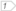#Boost C++ Libraries

...one of the most highly regarded and expertly designed C++ library projects in the world.

##### length (with strategy)

Calculates the length of a geometry using the specified strategy.

###### Description

The free function length calculates the length (the sum of distances between consecutive points) of a geometry using the specified strategy. Reasons to specify a strategy include: use another coordinate system for calculations; construct the strategy beforehand (e.g. with the radius of the Earth); select a strategy when there are more than one available for a calculation.

###### Synopsis

```template<typename Geometry, typename Strategy>
default_length_result<Geometry>::type length(Geometry const & geometry, Strategy const & strategy)```

###### Parameters

Type

Concept

Name

Description

Geometry const &

Any type fulfilling a Geometry Concept

geometry

A model of the specified concept

Strategy const &

Any type fulfilling a distance Strategy Concept

strategy

The strategy which will be used for distance calculations

###### Returns

The calculated length

Either

`#include <boost/geometry.hpp>`

Or

`#include <boost/geometry/algorithms/length.hpp>`

###### Conformance

The function length implements function Length from the OGC Simple Feature Specification.

###### Behavior

Case

Behavior

pointlike (e.g. point)

Returns 0

linear (e.g. linestring)

Returns the length

areal (e.g. polygon)

Returns 0

Linear

###### Examples

The following example shows the length measured over a sphere, expressed in kilometers. To do that the radius of the sphere must be specified in the constructor of the strategy.

```#include <iostream>
#include <boost/geometry.hpp>
#include <boost/geometry/geometries/linestring.hpp>

int main()
{
using namespace boost::geometry;
typedef model::point<float, 2, cs::spherical_equatorial<degree> > P;
model::linestring<P> line;
line.push_back(P(2, 41));
line.push_back(P(2, 48));
line.push_back(P(5, 52));
double const mean_radius = 6371.0;std::cout << "length is "
```length is 1272.03 kilometers